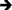## ▼ <= Less Than or Equals

Tag Link Category <= Less Than or Equals Symbol Symbol No Preferred 5.0 Unchanged Any Boolean None LCAPI Lasso 8.5, Lasso 8.0, Lasso 7.0, Lasso 6.0, Lasso 5.0

## ▼ Description

<= checks that the left parameter is less than or equal to the right parameter. It compares either numbers or strings (which are checked alphabetically).

Note: If the parameter on the right hand side of the symbol is a negative literal it should be surrounded by parentheses. For example, (4 <= (-1)).

## ▼ Syntax

[If: Left_Parameter <= Right_Parameter]
...
[/If]

<?LassoScript
If: Left_Parameter <= Right_Parameter;
...
/If;
?>

## ▼ Parameters

Required Parameters
Left_Parameter The value to be compared.
Right_Parameter The value to be compared.

## ▼ Examples

To return a result if one value is less than or equal to another:

Use the less than or equals symbol <= within an opening [If] tag. The following example checks if 100 is less than or equal to 100.5. The contents of the [If] ... [/If] tags is returned since the condition is True.

[If: 100 <= 100.5]
The integer value is less than or equal to the decimal value.
[/If]The integer value is less than or equal to the decimal value.